Specific Gravity Determination - Pycnometer Method

Specific gravity of solids is defined as weight of solids in a given volume, to the weight of equivalent volume of water.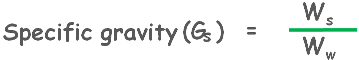We can determine the value of specific gravity using the pycnometer bottle. First we weigh the empty bottle and note weight as w1.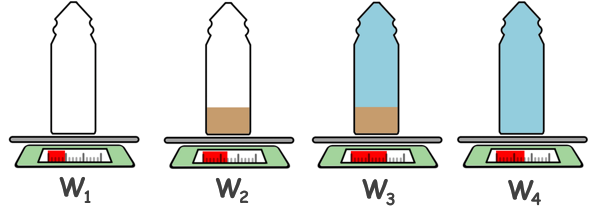Then we take some 300gm of soil which has been previously dried in oven, which means all of its water has been taken out. Put this sample into pycnometer and weigh it to note down weight w2.

Next we fill the pycnometer with water completely up to the top and stir the sample with glass rod to ensure that all the entrapped air was removed. The sample is weighed and now noted as w3.

Finally pycnometer is emptied and cleaned and filled with only water and its weight is noted as w4.

Now as we know that specific gravity of solids is weight of solids to the weight of same volume of water. From our observed weights we can compute these weight of solids and weight of water values.

Weight of solids will be weight of dry soil as there is no water in the sample so weight of water is zero and weight of air is considered as zero. So weight of dry soil can be calculated by removing weight of empty bottle W1 from the weight of dry soil + bottle W2.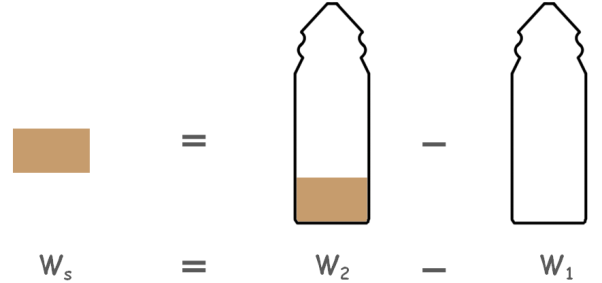Ws = W2 - W1                 ....(1)

Now we need to calculate the weight of water which has the same volume as that of dry soil.

See that from weight W3 (weight of bottle + dry soil + water) if we remove W4 (weight of bottle + total water) we will be left with weight of dry soil and weight of water which has the same volume as that of dry soil.W3 - W4 = Ws - Ww

Now here from equation number 1 we can write weight of dry soil as W2 – W1.So we can have the weight of water as

Ww = W2 - W1 - (W3 - W4)

substitute these values in the specific gravity equation and we can have the specific gravity of solids present in our soil sample.Specific gravity values in soil engineering, unless specified, are usually reported at 27 degree centigrade temperature, which is considered as room temperature. So if test was performed at T degree centigrade the specific gravity at 27 degree can be calculated by this formula.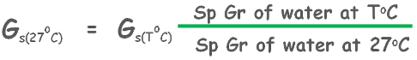which can also be written as this: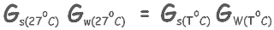Tags : pycnometer, specific gravity, soil test

Published on :2019-09-06